# RS Aggarwal Solutions for Class 9 Chapter 9: Congruence of Triangles and Inequalities in a Triangle Exercise 9B

## RS Aggarwal Solutions for Class 9 Maths Exercise 9B PDF

RS Aggarwal Solutions are prepared in order to make the concepts easy to learn for the students. While solving the textbook exercise problems, the students can make use of the PDF which is available. Vast research is conducted on the topics by subject experts to improve concept based approach while solving problems. The exercise wise solutions for each chapter are prepared based on the CBSE guidelines. It is helpful for the students in order to complete the whole syllabus accordingly. RS Aggarwal Solutions for Class 9 Maths Chapter 9 Congruence of Triangles and Inequalities in a Triangle Exercise 9B are provided here.

## RS Aggarwal Solutions for Class 9 Chapter 9: Congruence of Triangles and Inequalities in a Triangle Exercise 9B Download PDF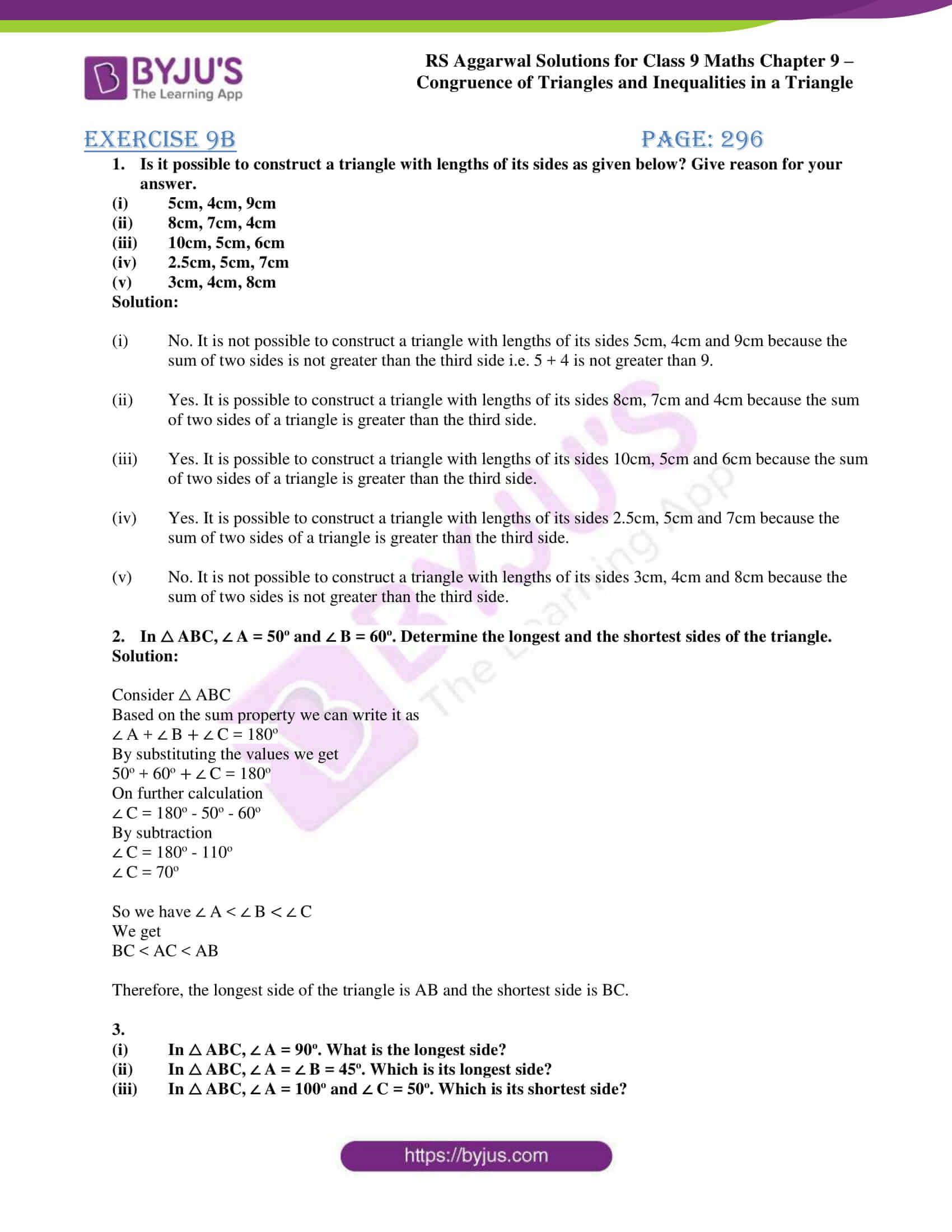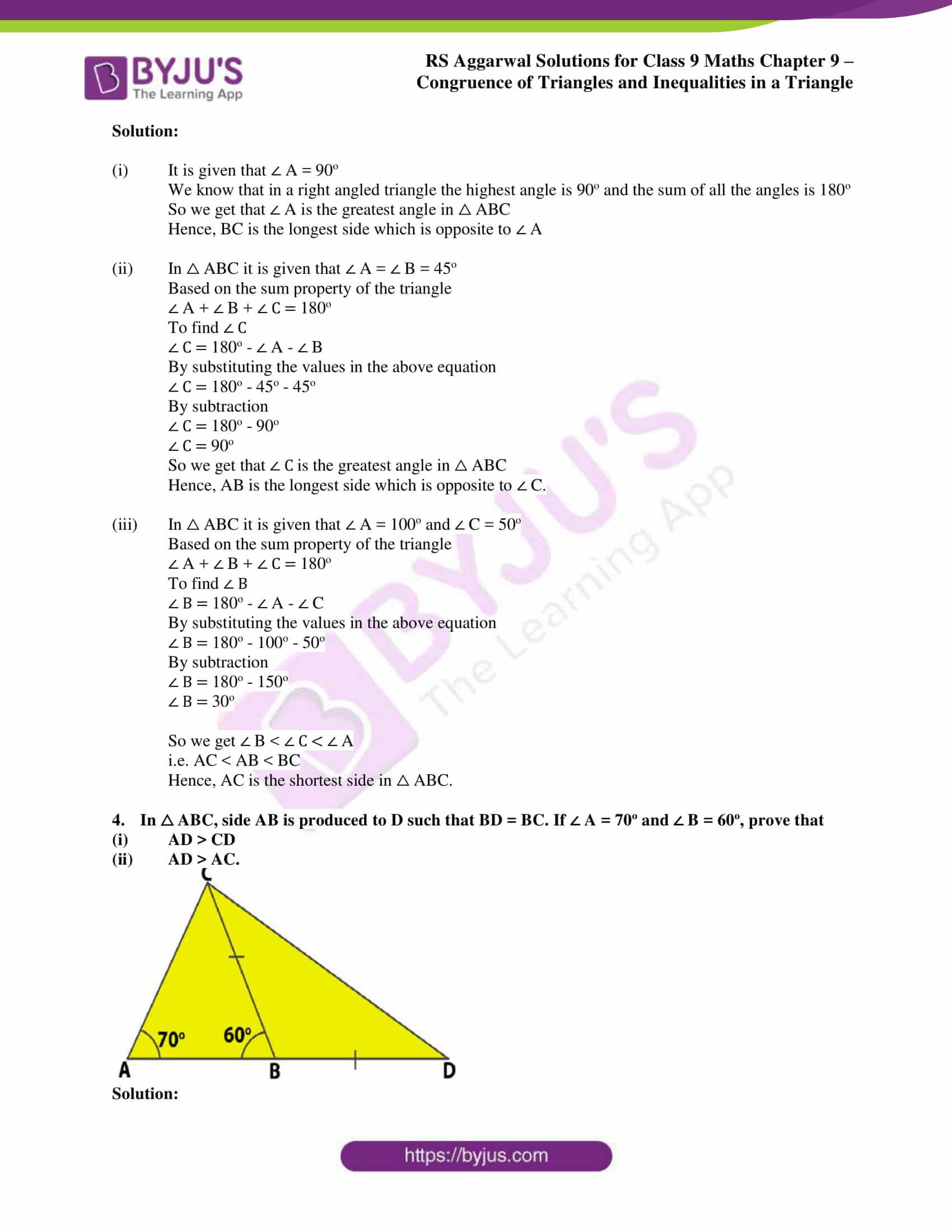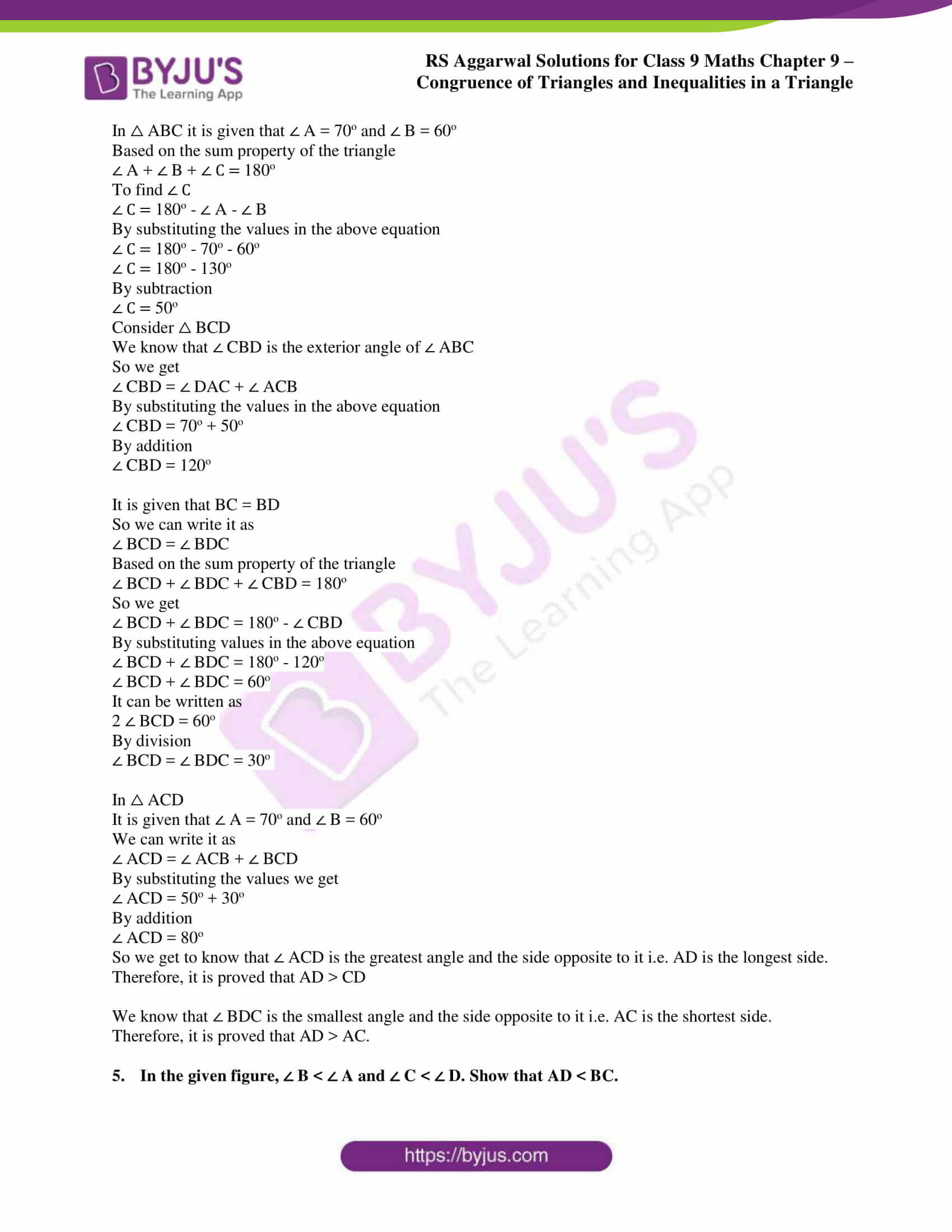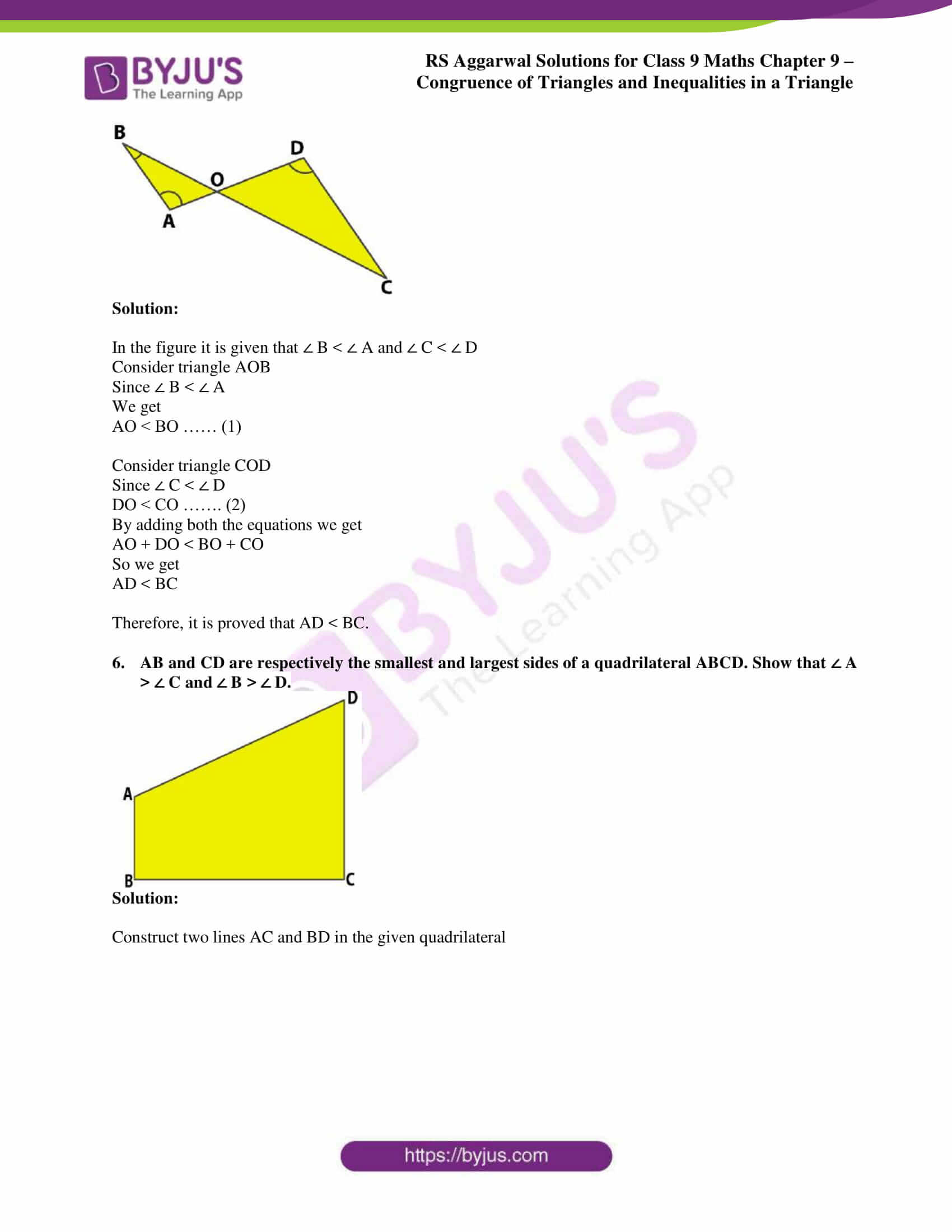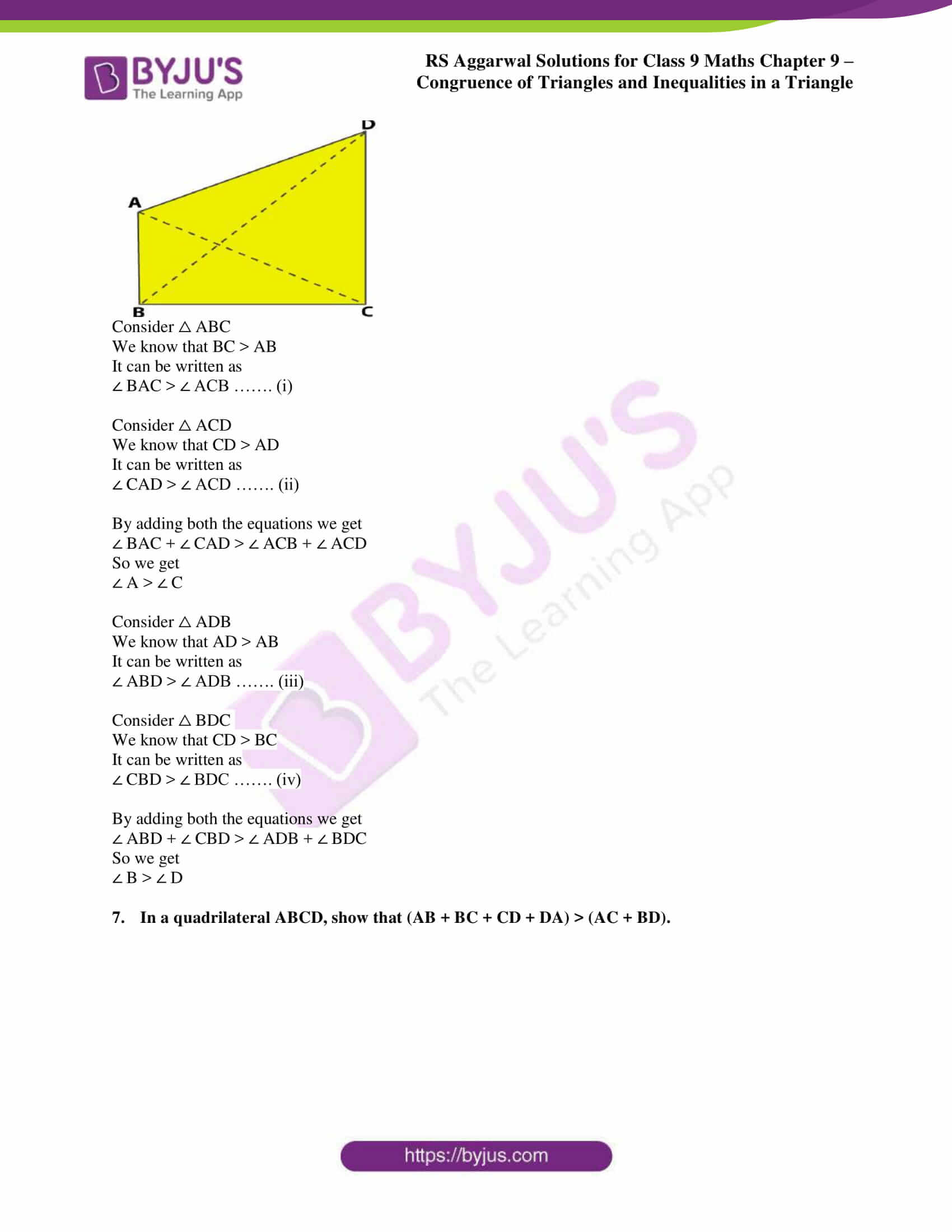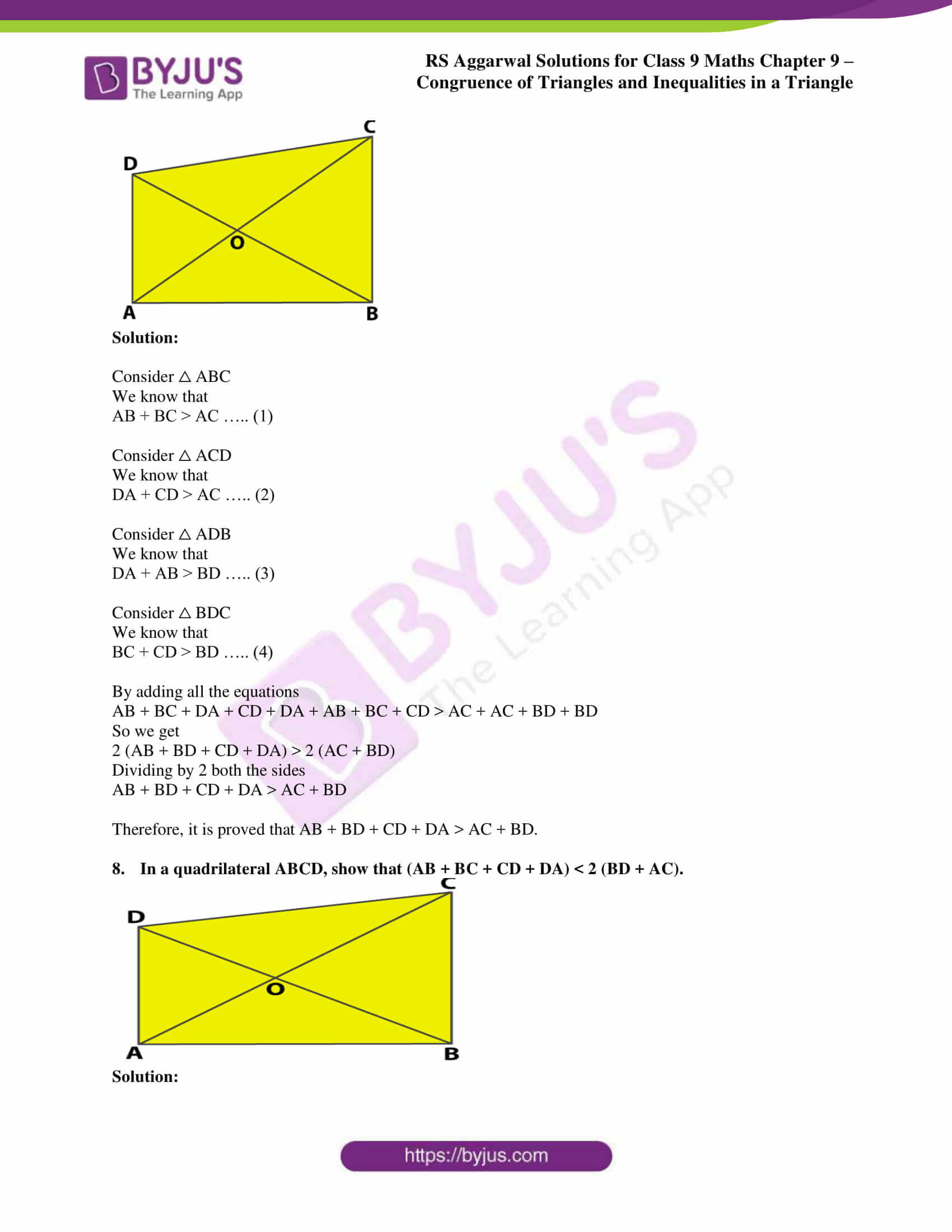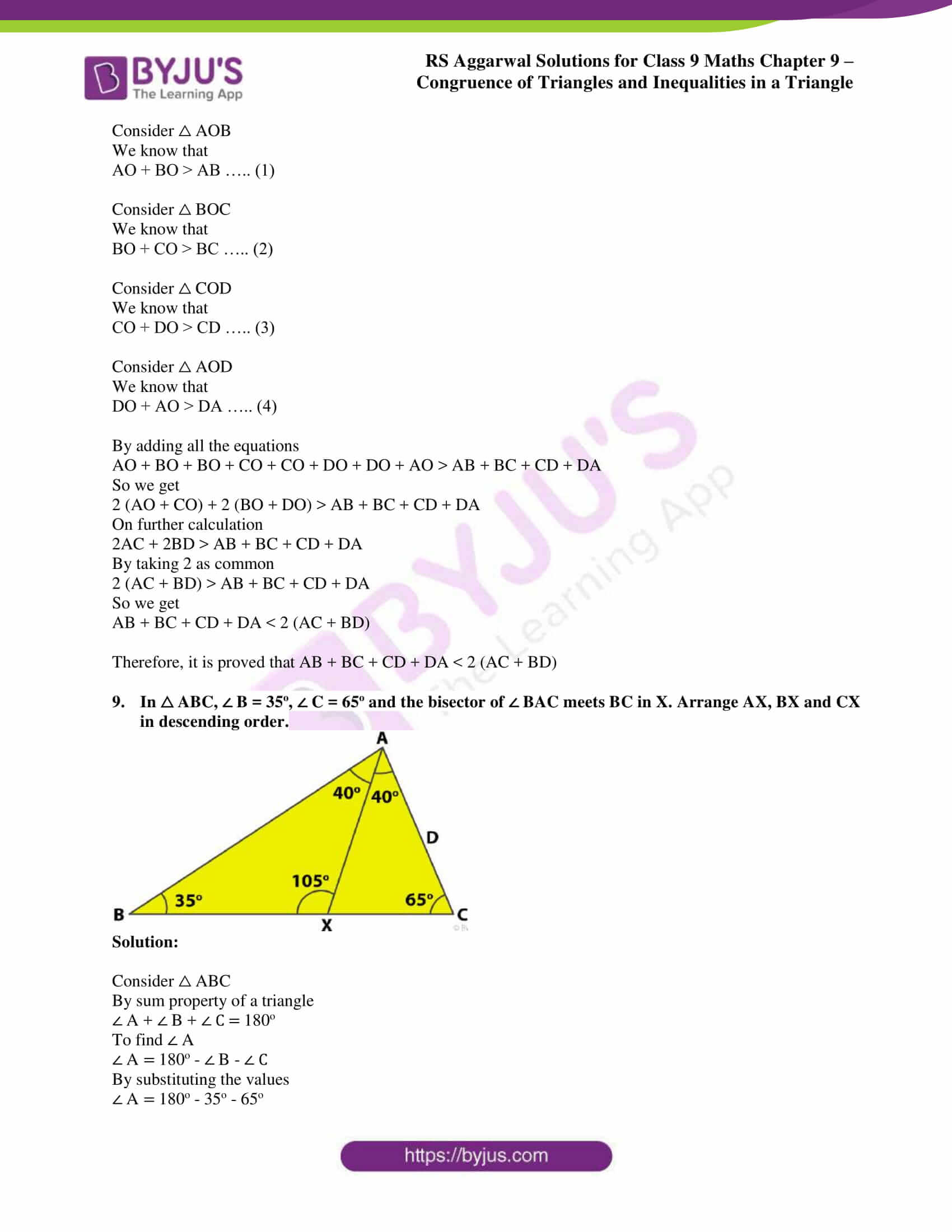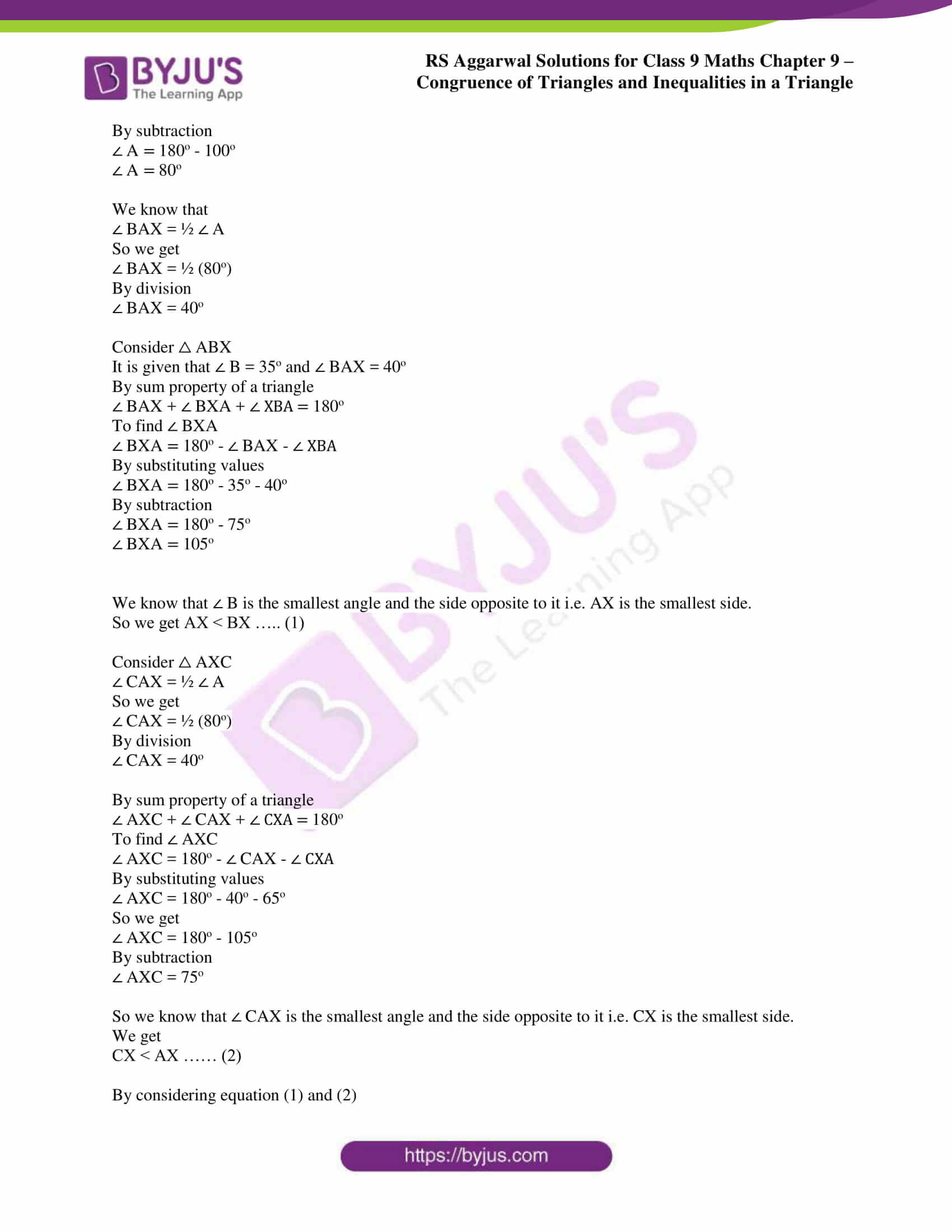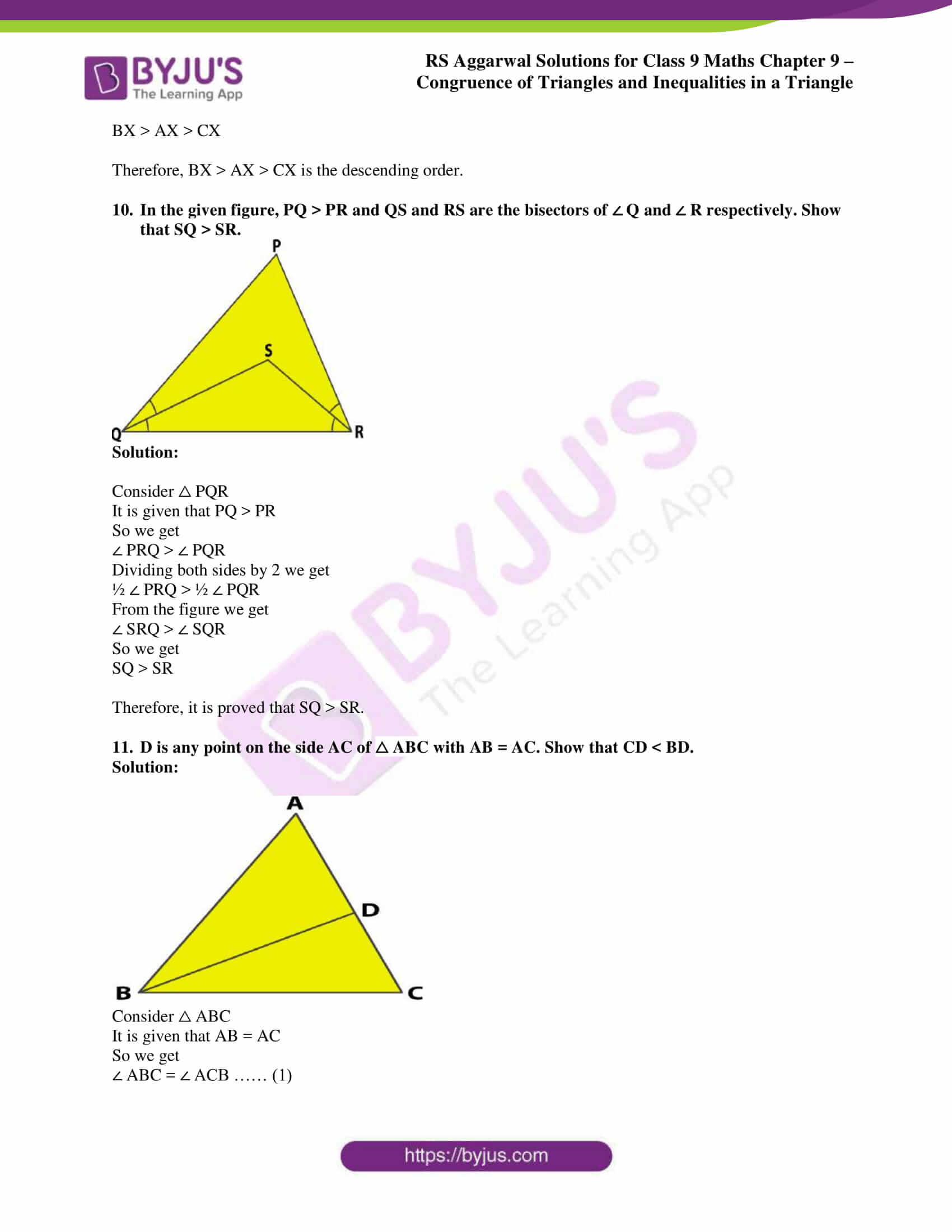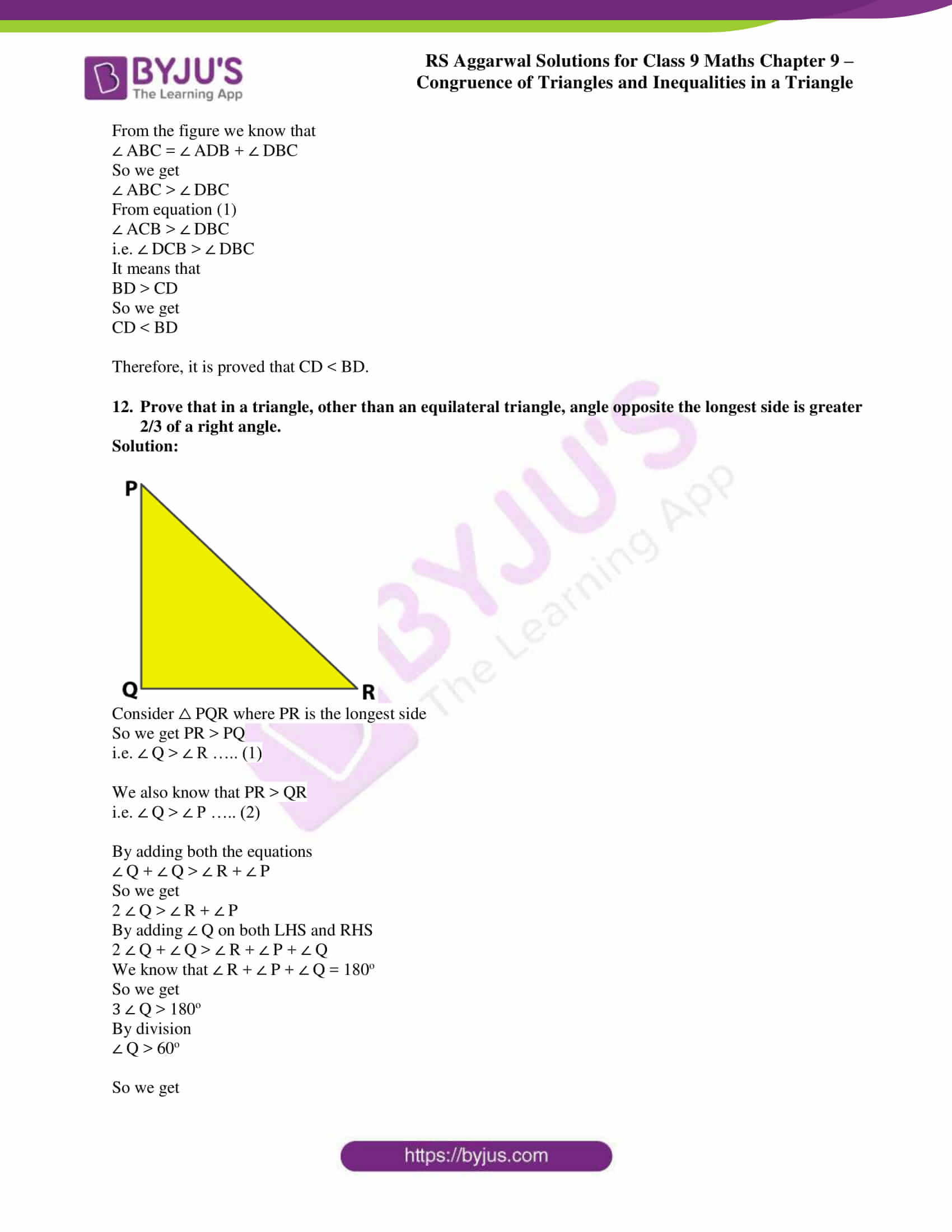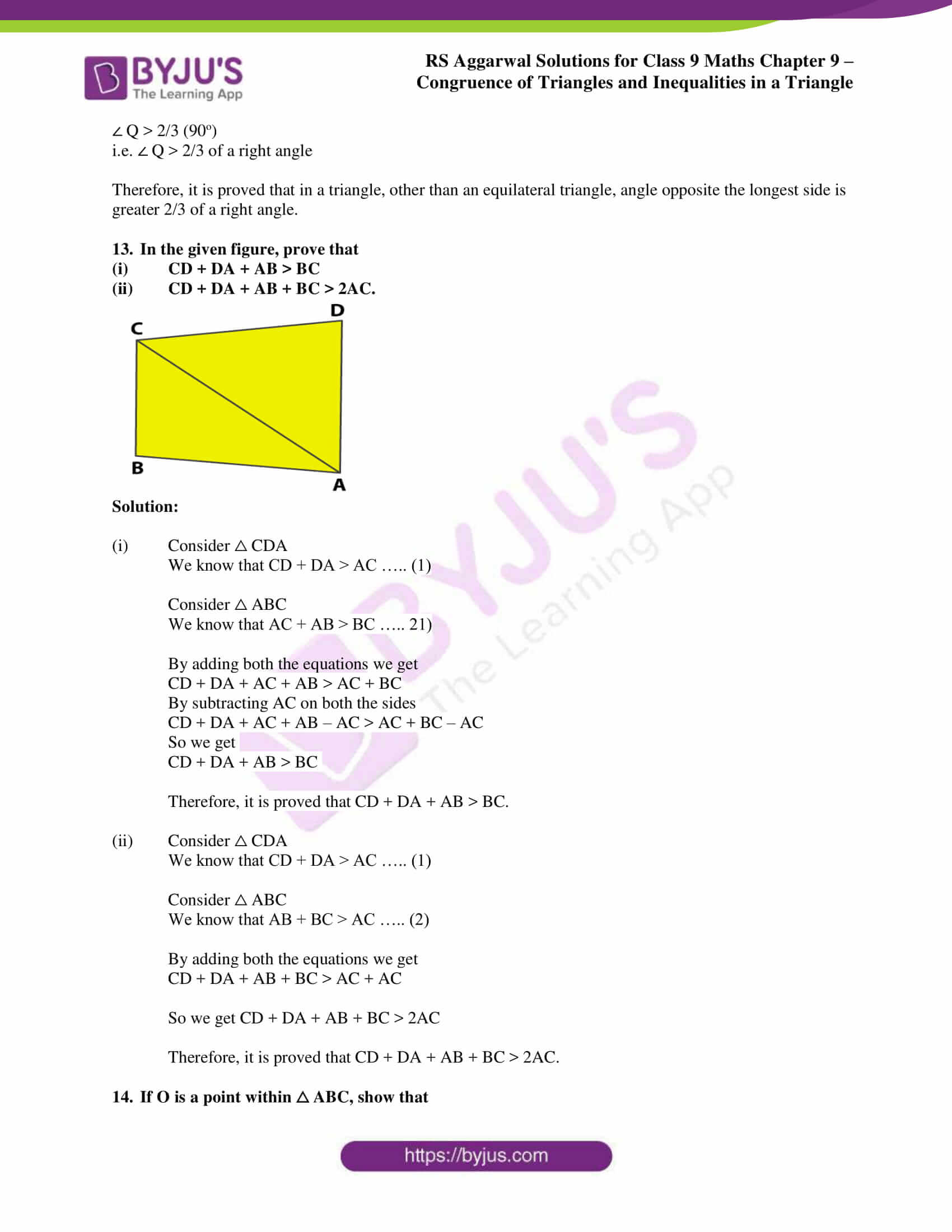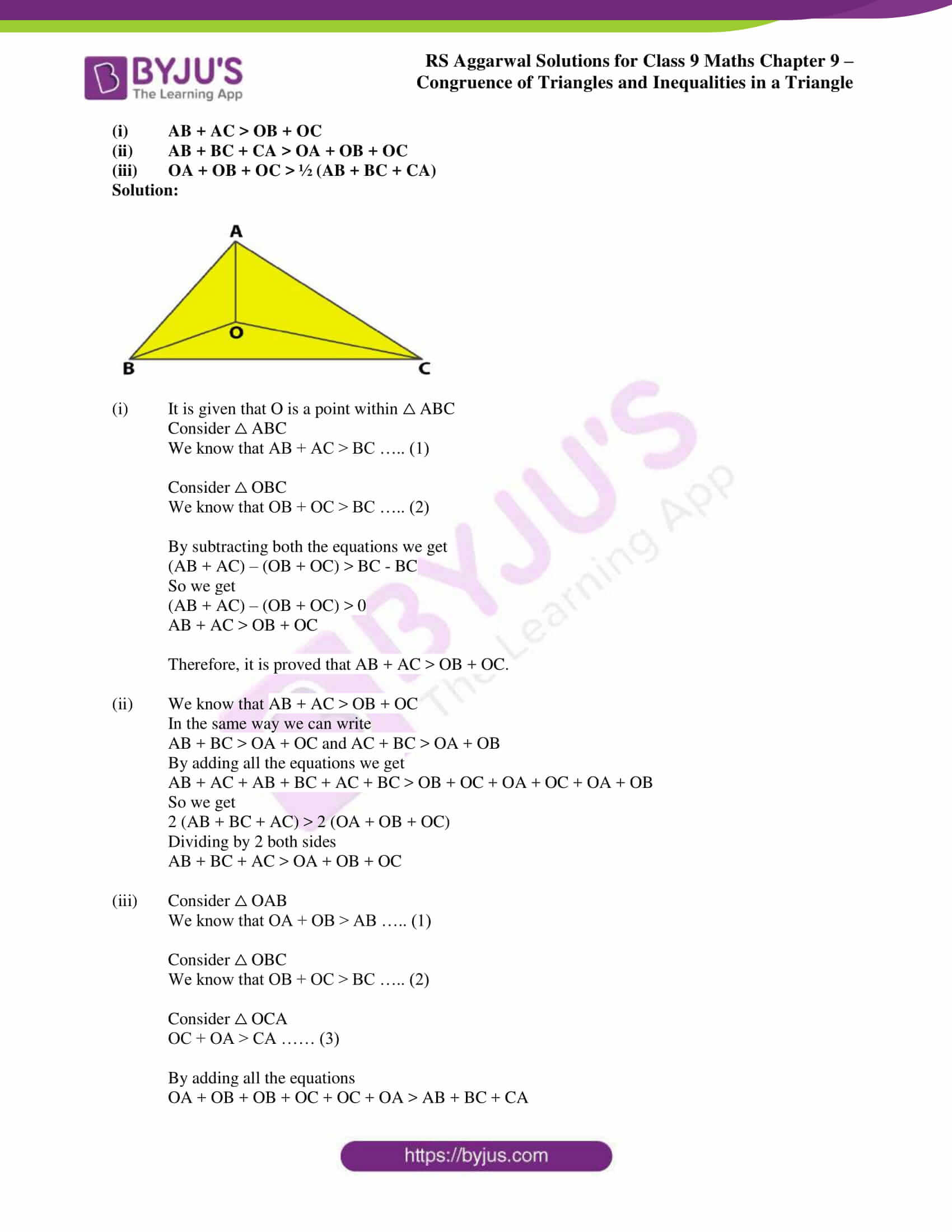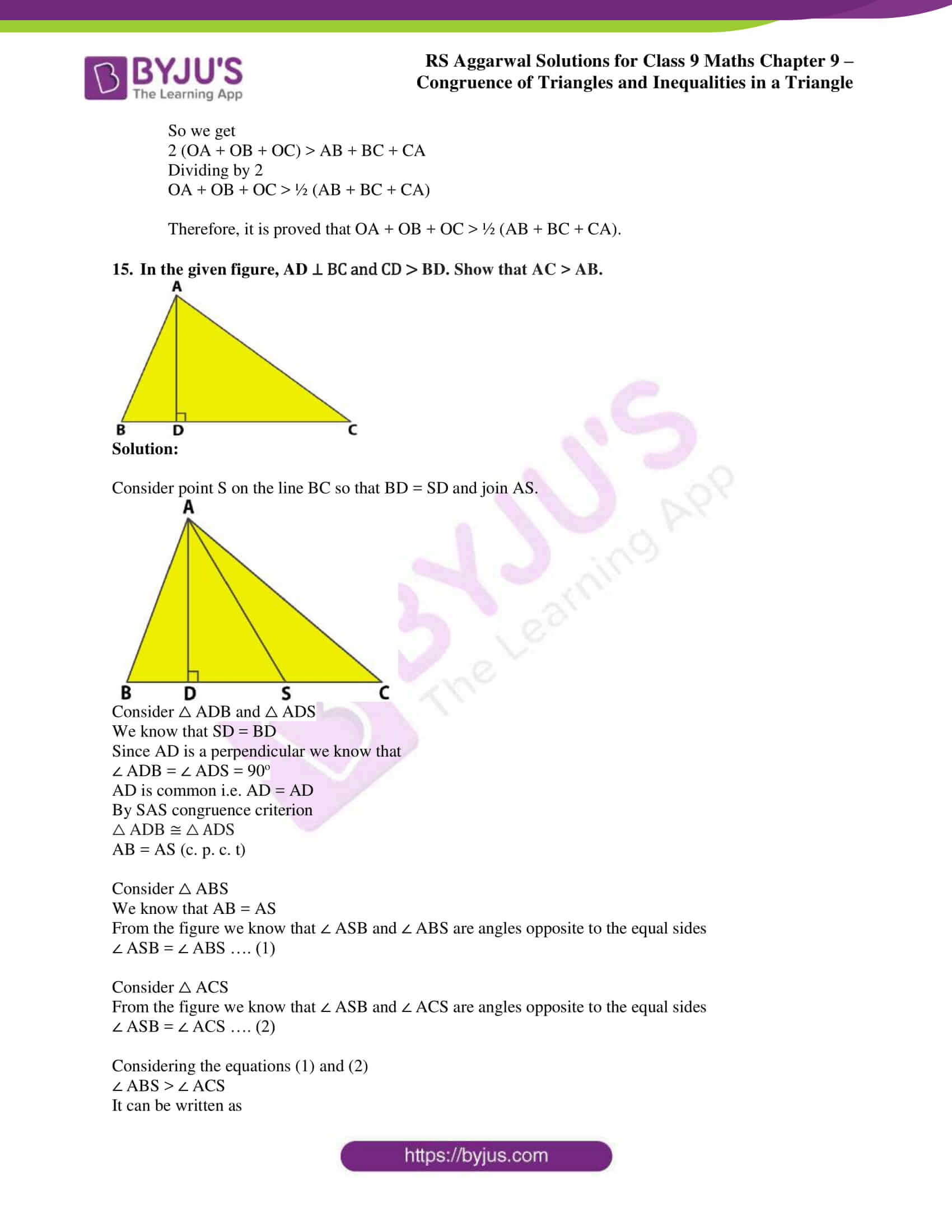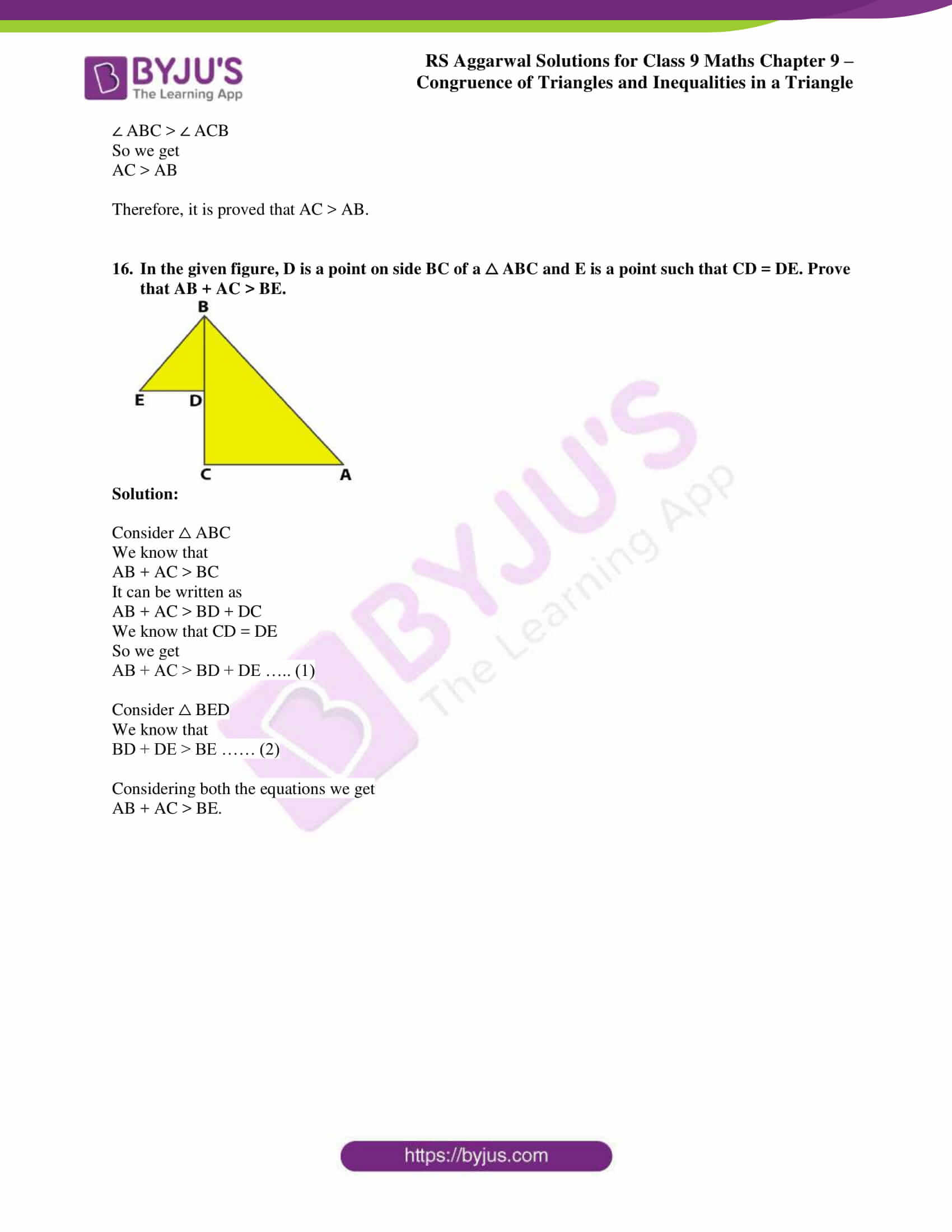## Access RS Aggarwal Solutions for Class 9 Chapter 9: Congruence of Triangles and Inequalities in a Triangle Exercise 9B

1. Is it possible to construct a triangle with lengths of its sides as given below? Give reason for your answer.

(i) 5cm, 4cm, 9cm

(ii) 8cm, 7cm, 4cm

(iii) 10cm, 5cm, 6cm

(iv) 2.5cm, 5cm, 7cm

(v) 3cm, 4cm, 8cm

Solution:

(i) No. It is not possible to construct a triangle with lengths of its sides 5cm, 4cm and 9cm because the sum of two sides is not greater than the third side i.e. 5 + 4 is not greater than 9.

(ii) Yes. It is possible to construct a triangle with lengths of its sides 8cm, 7cm and 4cm because the sum of two sides of a triangle is greater than the third side.

(iii) Yes. It is possible to construct a triangle with lengths of its sides 10cm, 5cm and 6cm because the sum of two sides of a triangle is greater than the third side.

(iv) Yes. It is possible to construct a triangle with lengths of its sides 2.5cm, 5cm and 7cm because the sum of two sides of a triangle is greater than the third side.

(v) No. It is not possible to construct a triangle with lengths of its sides 3cm, 4cm and 8cm because the sum of two sides is not greater than the third side.

2. In △ ABC, ∠ A = 50o and ∠ B = 60o. Determine the longest and the shortest sides of the triangle.

Solution:

Consider △ ABC

Based on the sum property we can write it as

∠ A + ∠ B + ∠ C = 180o

By substituting the values we get

50o + 60o + ∠ C = 180o

On further calculation

∠ C = 180o – 50o – 60o

By subtraction

∠ C = 180o – 110o

∠ C = 70o

So we have ∠ A < ∠ B < ∠ C

We get

BC < AC < AB

Therefore, the longest side of the triangle is AB and the shortest side is BC.

3.

(i) In △ ABC, ∠ A = 90o. What is the longest side?

(ii) In △ ABC, ∠ A = ∠ B = 45o. Which is its longest side?

(iii) In △ ABC, ∠ A = 100o and ∠ C = 50o. Which is its shortest side?

Solution:

(i) It is given that ∠ A = 90o

We know that in a right angled triangle the highest angle is 90o and the sum of all the angles is 180o

So we get that ∠ A is the greatest angle in △ ABC

Hence, BC is the longest side which is opposite to ∠ A

(ii) In △ ABC it is given that ∠ A = ∠ B = 45o

Based on the sum property of the triangle

∠ A + ∠ B + ∠ C = 180o

To find ∠ C

∠ C = 180o – ∠ A – ∠ B

By substituting the values in the above equation

∠ C = 180o – 45o – 45o

By subtraction

∠ C = 180o – 90o

∠ C = 90o

So we get that ∠ C is the greatest angle in △ ABC

Hence, AB is the longest side which is opposite to ∠ C.

(iii) In △ ABC it is given that ∠ A = 100o and ∠ C = 50o

Based on the sum property of the triangle

∠ A + ∠ B + ∠ C = 180o

To find ∠ B

∠ B = 180o – ∠ A – ∠ C

By substituting the values in the above equation

∠ B = 180o – 100o – 50o

By subtraction

∠ B = 180o – 150o

∠ B = 30o

So we get ∠ B < ∠ C < ∠ A

i.e. AC < AB < BC

Hence, AC is the shortest side in △ ABC.

4. In △ ABC, side AB is produced to D such that BD = BC. If ∠ A = 70o and ∠ B = 60o, prove that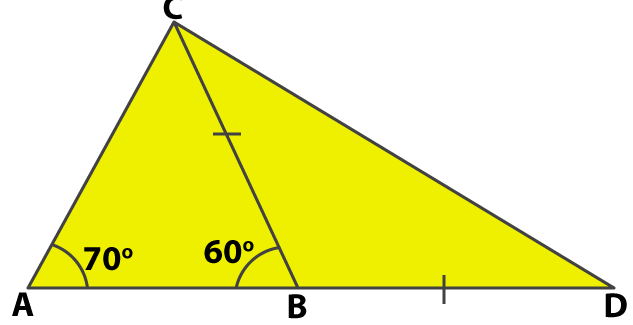Solution:

In △ ABC it is given that ∠ A = 70o and ∠ B = 60o

Based on the sum property of the triangle

∠ A + ∠ B + ∠ C = 180o

To find ∠ C

∠ C = 180o – ∠ A – ∠ B

By substituting the values in the above equation

∠ C = 180o – 70o – 60o

∠ C = 180o – 130o

By subtraction

∠ C = 50o

Consider △ BCD

We know that ∠ CBD is the exterior angle of ∠ ABC

So we get

∠ CBD = ∠ DAC + ∠ ACB

By substituting the values in the above equation

∠ CBD = 70o + 50o

∠ CBD = 120o

It is given that BC = BD

So we can write it as

∠ BCD = ∠ BDC

Based on the sum property of the triangle

∠ BCD + ∠ BDC + ∠ CBD = 180o

So we get

∠ BCD + ∠ BDC = 180o – ∠ CBD

By substituting values in the above equation

∠ BCD + ∠ BDC = 180o – 120o

∠ BCD + ∠ BDC = 60o

It can be written as

2 ∠ BCD = 60o

By division

∠ BCD = ∠ BDC = 30o

In △ ACD

It is given that ∠ A = 70o and ∠ B = 60o

We can write it as

∠ ACD = ∠ ACB + ∠ BCD

By substituting the values we get

∠ ACD = 50o + 30o

∠ ACD = 80o

So we get to know that ∠ ACD is the greatest angle and the side opposite to it i.e. AD is the longest side.

Therefore, it is proved that AD > CD

We know that ∠ BDC is the smallest angle and the side opposite to it i.e. AC is the shortest side.

Therefore, it is proved that AD > AC.

5. In the given figure, ∠ B < ∠ A and ∠ C < ∠ D. Show that AD < BC.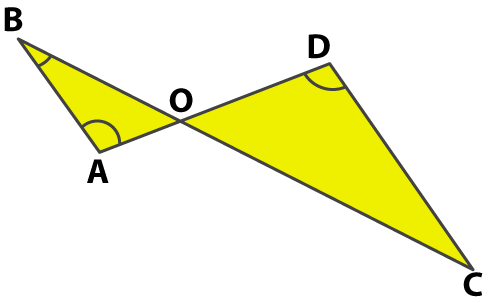Solution:

In the figure it is given that ∠ B < ∠ A and ∠ C < ∠ D

Consider triangle AOB

Since ∠ B < ∠ A

We get

AO < BO …… (1)

Consider triangle COD

Since ∠ C < ∠ D

DO < CO ……. (2)

By adding both the equations we get

AO + DO < BO + CO

So we get

Therefore, it is proved that AD < BC.

6. AB and CD are respectively the smallest and largest sides of a quadrilateral ABCD. Show that ∠ A > ∠ C and ∠ B > ∠ D.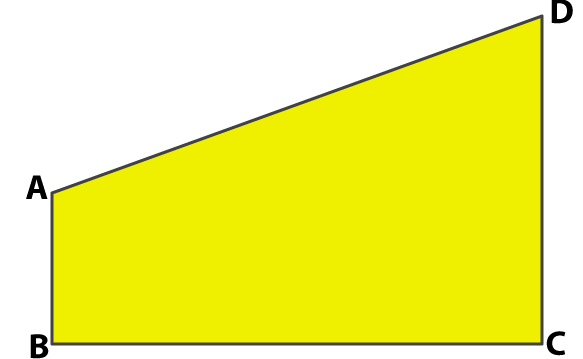Solution:

Construct two lines AC and BD in the given quadrilateral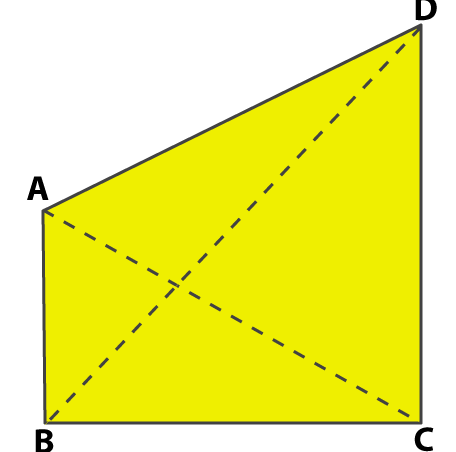Consider △ ABC

We know that BC > AB

It can be written as

∠ BAC > ∠ ACB ……. (i)

Consider △ ACD

We know that CD > AD

It can be written as

∠ CAD > ∠ ACD ……. (ii)

By adding both the equations we get

∠ BAC + ∠ CAD > ∠ ACB + ∠ ACD

So we get

∠ A > ∠ C

We know that AD > AB

It can be written as

∠ ABD > ∠ ADB ……. (iii)

Consider △ BDC

We know that CD > BC

It can be written as

∠ CBD > ∠ BDC ……. (iv)

By adding both the equations we get

∠ ABD + ∠ CBD > ∠ ADB + ∠ BDC

So we get

∠ B > ∠ D

7. In a quadrilateral ABCD, show that (AB + BC + CD + DA) > (AC + BD).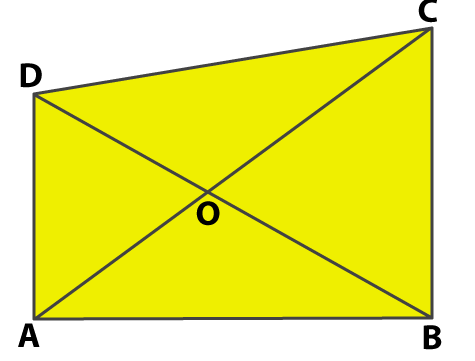Solution:

Consider △ ABC

We know that

AB + BC > AC ….. (1)

Consider △ ACD

We know that

DA + CD > AC ….. (2)

We know that

DA + AB > BD ….. (3)

Consider △ BDC

We know that

BC + CD > BD ….. (4)

AB + BC + DA + CD + DA + AB + BC + CD > AC + AC + BD + BD

So we get

2 (AB + BD + CD + DA) > 2 (AC + BD)

Dividing by 2 both the sides

AB + BD + CD + DA > AC + BD

Therefore, it is proved that AB + BD + CD + DA > AC + BD.

8. In a quadrilateral ABCD, show that (AB + BC + CD + DA) < 2 (BD + AC).Solution:

Consider △ AOB

We know that

AO + BO > AB ….. (1)

Consider △ BOC

We know that

BO + CO > BC ….. (2)

Consider △ COD

We know that

CO + DO > CD ….. (3)

Consider △ AOD

We know that

DO + AO > DA ….. (4)

AO + BO + BO + CO + CO + DO + DO + AO > AB + BC + CD + DA

So we get

2 (AO + CO) + 2 (BO + DO) > AB + BC + CD + DA

On further calculation

2AC + 2BD > AB + BC + CD + DA

By taking 2 as common

2 (AC + BD) > AB + BC + CD + DA

So we get

AB + BC + CD + DA < 2 (AC + BD)

Therefore, it is proved that AB + BC + CD + DA < 2 (AC + BD)

9. In △ ABC, ∠ B = 35o, ∠ C = 65o and the bisector of ∠ BAC meets BC in X. Arrange AX, BX and CX in descending order.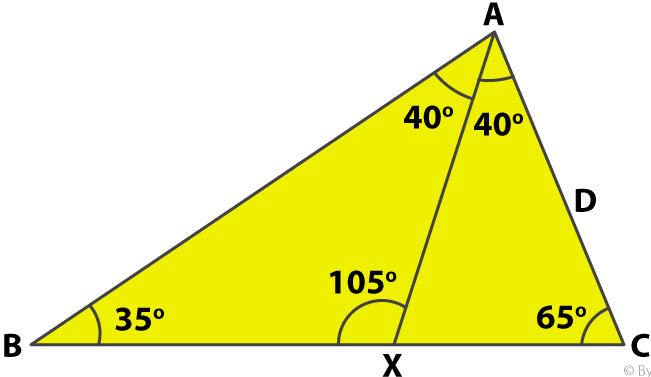Solution:

Consider △ ABC

By sum property of a triangle

∠ A + ∠ B + ∠ C = 180o

To find ∠ A

∠ A = 180o – ∠ B – ∠ C

By substituting the values

∠ A = 180o – 35o – 65o

By subtraction

∠ A = 180o – 100o

∠ A = 80o

We know that

∠ BAX = ½ ∠ A

So we get

∠ BAX = ½ (80o)

By division

∠ BAX = 40o

Consider △ ABX

It is given that ∠ B = 35o and ∠ BAX = 40o

By sum property of a triangle

∠ BAX + ∠ BXA + ∠ XBA = 180o

To find ∠ BXA

∠ BXA = 180o – ∠ BAX – ∠ XBA

By substituting values

∠ BXA = 180o – 35o – 40o

By subtraction

∠ BXA = 180o – 75o

∠ BXA = 105o

We know that ∠ B is the smallest angle and the side opposite to it i.e. AX is the smallest side.

So we get AX < BX ….. (1)

Consider △ AXC

∠ CAX = ½ ∠ A

So we get

∠ CAX = ½ (80o)

By division

∠ CAX = 40o

By sum property of a triangle

∠ AXC + ∠ CAX + ∠ CXA = 180o

To find ∠ AXC

∠ AXC = 180o – ∠ CAX – ∠ CXA

By substituting values

∠ AXC = 180o – 40o – 65o

So we get

∠ AXC = 180o – 105o

By subtraction

∠ AXC = 75o

So we know that ∠ CAX is the smallest angle and the side opposite to it i.e. CX is the smallest side.

We get

CX < AX …… (2)

By considering equation (1) and (2)

BX > AX > CX

Therefore, BX > AX > CX is the descending order.

10. In the given figure, PQ > PR and QS and RS are the bisectors of ∠ Q and ∠ R respectively. Show that SQ > SR.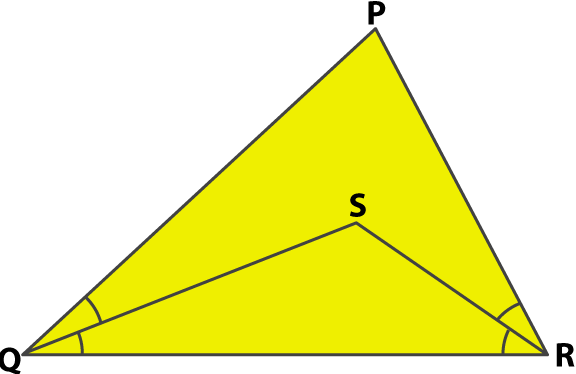Solution:

Consider △ PQR

It is given that PQ > PR

So we get

∠ PRQ > ∠ PQR

Dividing both sides by 2 we get

½ ∠ PRQ > ½ ∠ PQR

From the figure we get

∠ SRQ > ∠ SQR

So we get

SQ > SR

Therefore, it is proved that SQ > SR.

11. D is any point on the side AC of △ ABC with AB = AC. Show that CD < BD.

Solution: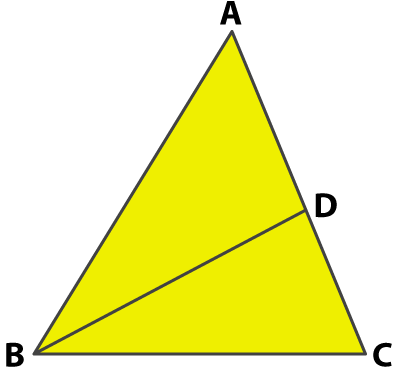Consider △ ABC

It is given that AB = AC

So we get

∠ ABC = ∠ ACB …… (1)

From the figure we know that

∠ ABC = ∠ ADB + ∠ DBC

So we get

∠ ABC > ∠ DBC

From equation (1)

∠ ACB > ∠ DBC

i.e. ∠ DCB > ∠ DBC

It means that

BD > CD

So we get

CD < BD

Therefore, it is proved that CD < BD.

12. Prove that in a triangle, other than an equilateral triangle, angle opposite the longest side is greater 2/3 of a right angle.

Solution: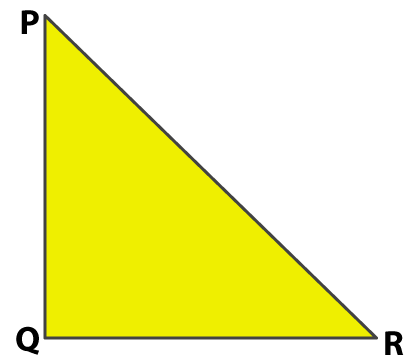Consider △ PQR where PR is the longest side

So we get PR > PQ

i.e. ∠ Q > ∠ R ….. (1)

We also know that PR > QR

i.e. ∠ Q > ∠ P ….. (2)

∠ Q + ∠ Q > ∠ R + ∠ P

So we get

2 ∠ Q > ∠ R + ∠ P

By adding ∠ Q on both LHS and RHS

2 ∠ Q + ∠ Q > ∠ R + ∠ P + ∠ Q

We know that ∠ R + ∠ P + ∠ Q = 180o

So we get

3 ∠ Q > 180o

By division

∠ Q > 60o

So we get

∠ Q > 2/3 (90o)

i.e. ∠ Q > 2/3 of a right angle

Therefore, it is proved that in a triangle, other than an equilateral triangle, angle opposite the longest side is greater 2/3 of a right angle.

13. In the given figure, prove that

(i) CD + DA + AB > BC

(ii) CD + DA + AB + BC > 2AC.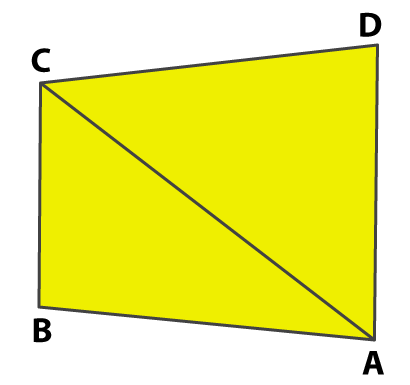Solution:

(i) Consider △ CDA

We know that CD + DA > AC ….. (1)

Consider △ ABC

We know that AC + AB > BC ….. 21)

By adding both the equations we get

CD + DA + AC + AB > AC + BC

By subtracting AC on both the sides

CD + DA + AC + AB – AC > AC + BC – AC

So we get

CD + DA + AB > BC

Therefore, it is proved that CD + DA + AB > BC.

(ii) Consider △ CDA

We know that CD + DA > AC ….. (1)

Consider △ ABC

We know that AB + BC > AC ….. (2)

By adding both the equations we get

CD + DA + AB + BC > AC + AC

So we get CD + DA + AB + BC > 2AC

Therefore, it is proved that CD + DA + AB + BC > 2AC.

14. If O is a point within △ ABC, show that

(i) AB + AC > OB + OC

(ii) AB + BC + CA > OA + OB + OC

(iii) OA + OB + OC > ½ (AB + BC + CA)

Solution: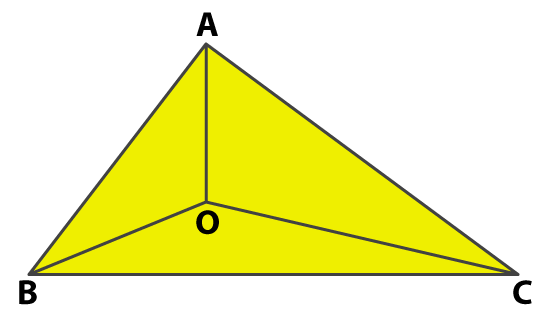(i) It is given that O is a point within △ ABC

Consider △ ABC

We know that AB + AC > BC ….. (1)

Consider △ OBC

We know that OB + OC > BC ….. (2)

By subtracting both the equations we get

(AB + AC) – (OB + OC) > BC – BC

So we get

(AB + AC) – (OB + OC) > 0

AB + AC > OB + OC

Therefore, it is proved that AB + AC > OB + OC.

(ii) We know that AB + AC > OB + OC

In the same way we can write

AB + BC > OA + OC and AC + BC > OA + OB

By adding all the equations we get

AB + AC + AB + BC + AC + BC > OB + OC + OA + OC + OA + OB

So we get

2 (AB + BC + AC) > 2 (OA + OB + OC)

Dividing by 2 both sides

AB + BC + AC > OA + OB + OC

(iii) Consider △ OAB

We know that OA + OB > AB ….. (1)

Consider △ OBC

We know that OB + OC > BC ….. (2)

Consider △ OCA

OC + OA > CA …… (3)

OA + OB + OB + OC + OC + OA > AB + BC + CA

So we get

2 (OA + OB + OC) > AB + BC + CA

Dividing by 2

OA + OB + OC > ½ (AB + BC + CA)

Therefore, it is proved that OA + OB + OC > ½ (AB + BC + CA).

15. In the given figure, AD ⊥ BC and CD > BD. Show that AC > AB.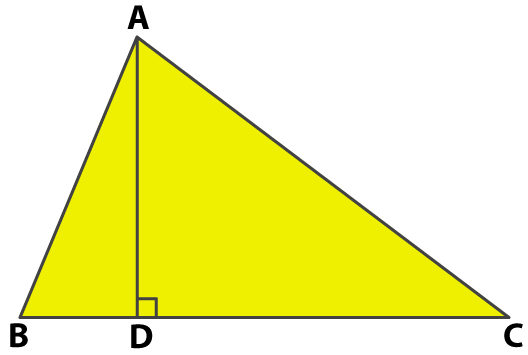Solution:

Consider point S on the line BC so that BD = SD and join AS.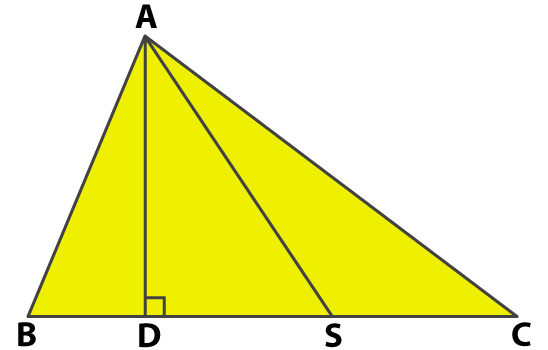We know that SD = BD

Since AD is a perpendicular we know that

By SAS congruence criterion

AB = AS (c. p. c. t)

Consider △ ABS

We know that AB = AS

From the figure we know that ∠ ASB and ∠ ABS are angles opposite to the equal sides

∠ ASB = ∠ ABS …. (1)

Consider △ ACS

From the figure we know that ∠ ASB and ∠ ACS are angles opposite to the equal sides

∠ ASB = ∠ ACS …. (2)

Considering the equations (1) and (2)

∠ ABS > ∠ ACS

It can be written as

∠ ABC > ∠ ACB

So we get

AC > AB

Therefore, it is proved that AC > AB.

16. In the given figure, D is a point on side BC of a △ ABC and E is a point such that CD = DE. Prove that AB + AC > BE.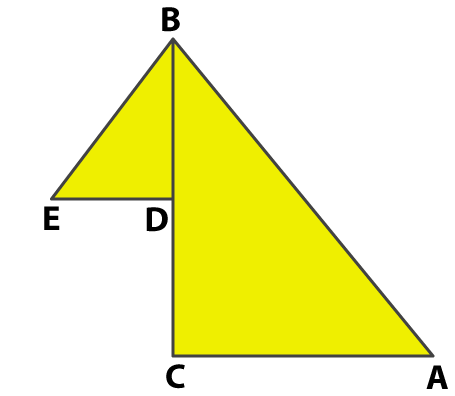Solution:

Consider △ ABC

We know that

AB + AC > BC

It can be written as

AB + AC > BD + DC

We know that CD = DE

So we get

AB + AC > BD + DE ….. (1)

Consider △ BED

We know that

BD + DE > BE …… (2)

Considering both the equations we get

AB + AC > BE.

### Access other exercise solutions of Class 9 Maths Chapter 9: Congruence of Triangles and Inequalities in a Triangle

Exercise 9A Solutions 27 Questions

### RS Aggarwal Solutions Class 9 Maths Chapter 9 – Congruence of Triangles and Inequalities in a Triangle Exercise 9B

RS Aggarwal Solutions Class 9 Maths Chapter 9 Congruence of Triangles and Inequalities in a Triangle Exercise 9B solves problems using theorems which are based on inequalities in a triangle.

### Key features of RS Aggarwal Solutions for Class 9 Maths Chapter 9: Congruence of Triangles and Inequalities in a Triangle Exercise 9B

• The solutions help students solve problems from Exercise 9B by gaining a better knowledge of concepts.
• Obtaining good marks in the board exams is made easier by using the solutions prepared by our subject experts.
• The answers are prepared according to the evaluation process which is followed in the CBSE board.
• The students can download the PDF and use it as a reference while solving exercise wise problems of RS Aggarwal textbook.